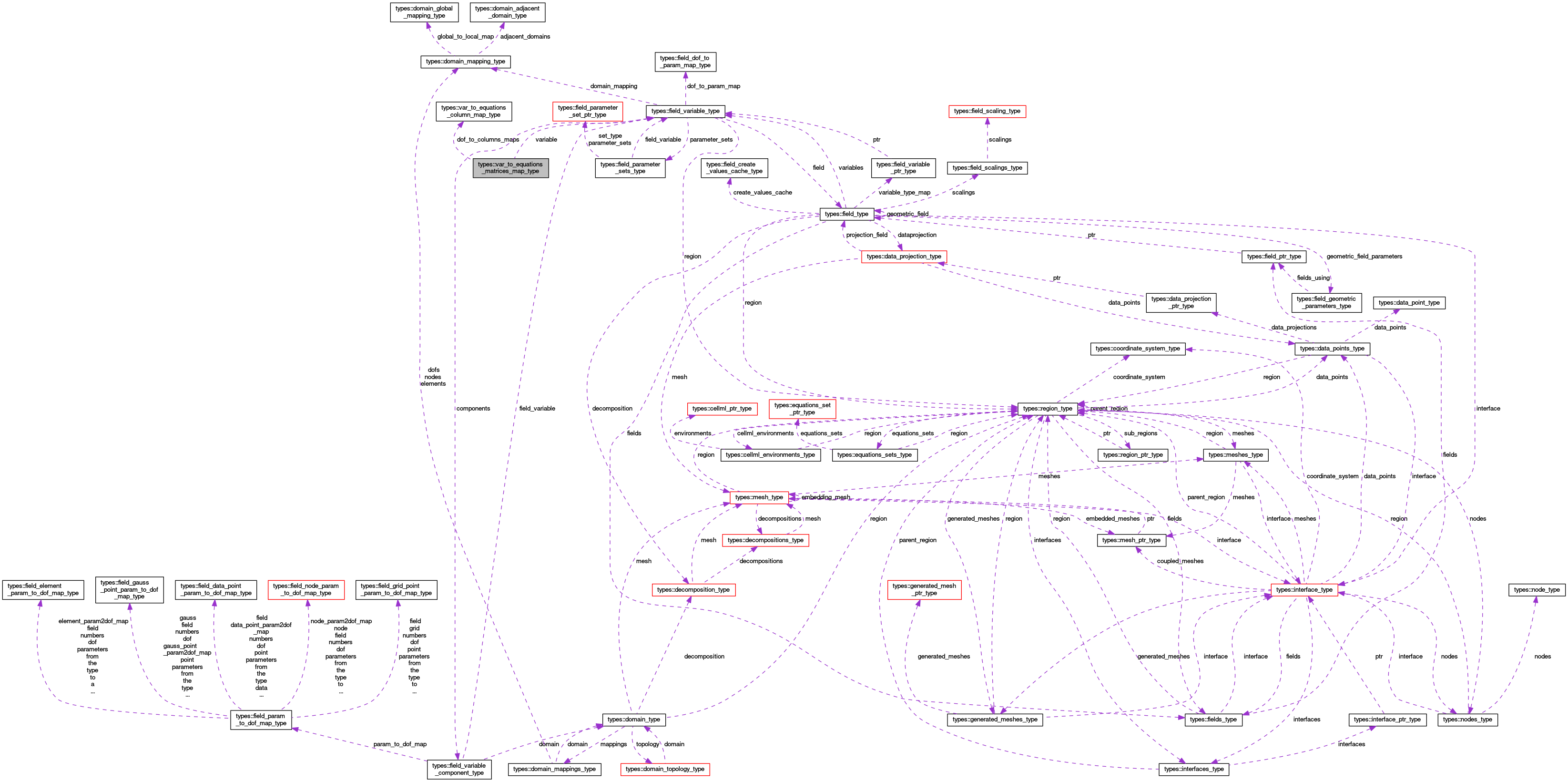OpenCMISS-Iron Internal API Documentation
types::var_to_equations_matrices_map_type Type Reference

Contains the mapping for a dependent variable type to the equations matrices. More...

Collaboration diagram for types::var_to_equations_matrices_map_type:[legend]

## Public Attributes

integer(intg) variable_index
The variable index for this variable to equations matrices map. More...

integer(intg) variable_type
The variable type for this variable to equations matrices map. More...

type(field_variable_type), pointer variable
A pointer to the field variable for this variable to equations matrices map. More...

integer(intg) number_of_equations_matrices
The number of equations matrices (linear or dynamic) this variable type is mapped to. If the number is -1 the variable is mapped to the RHS vector. If the number is zero then this variable type is not involved in the equations set and the rest of the type is not allocated. More...

integer(intg), dimension(:), allocatable equations_matrix_numbers
EQUATIONS_MATRIX_NUMBERS(i). The equations matrix number for the i'th matrix that this variable type is mapped to. More...

type(var_to_equations_column_map_type), dimension(:), allocatable dof_to_columns_maps
DOF_TO_COLUMNS_MAPS(i). The variable dof to equations columns for the i'th equations matrix. More...

integer(intg), dimension(:), allocatable dof_to_rows_map
DOF_TO_ROWS_MAP(dof_idx). The row number that the dof_idx'th variable dof is mapped to. More...

## Detailed Description

Contains the mapping for a dependent variable type to the equations matrices.

Definition at line 1548 of file types.f90.

## Member Data Documentation

 type(var_to_equations_column_map_type), dimension(:), allocatable types::var_to_equations_matrices_map_type::dof_to_columns_maps

DOF_TO_COLUMNS_MAPS(i). The variable dof to equations columns for the i'th equations matrix.

Definition at line 1554 of file types.f90.

 integer(intg), dimension(:), allocatable types::var_to_equations_matrices_map_type::dof_to_rows_map

DOF_TO_ROWS_MAP(dof_idx). The row number that the dof_idx'th variable dof is mapped to.

Definition at line 1555 of file types.f90.

 integer(intg), dimension(:), allocatable types::var_to_equations_matrices_map_type::equations_matrix_numbers

EQUATIONS_MATRIX_NUMBERS(i). The equations matrix number for the i'th matrix that this variable type is mapped to.

Definition at line 1553 of file types.f90.

 integer(intg) types::var_to_equations_matrices_map_type::number_of_equations_matrices

The number of equations matrices (linear or dynamic) this variable type is mapped to. If the number is -1 the variable is mapped to the RHS vector. If the number is zero then this variable type is not involved in the equations set and the rest of the type is not allocated.

Definition at line 1552 of file types.f90.

 type(field_variable_type), pointer types::var_to_equations_matrices_map_type::variable

A pointer to the field variable for this variable to equations matrices map.

Definition at line 1551 of file types.f90.

 integer(intg) types::var_to_equations_matrices_map_type::variable_index

The variable index for this variable to equations matrices map.

Definition at line 1549 of file types.f90.

 integer(intg) types::var_to_equations_matrices_map_type::variable_type

The variable type for this variable to equations matrices map.

Definition at line 1550 of file types.f90.